### IMO Shortlist 1985 problem 22

Kvaliteta:
Avg: 0.0
Težina:
Avg: 6.0
A circle with center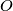$O$ passes through the vertices$A$ and$C$ of the triangle$ABC$ and intersects the segments$AB$ and$BC$ again at distinct points$K$ and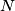$N$ respectively. Let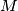$M$ be the point of intersection of the circumcircles of triangles$ABC$ and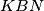$KBN$ (apart from$B$). Prove that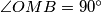$\angle OMB=90^{\circ}$.
Source: Međunarodna matematička olimpijada, shortlist 1985